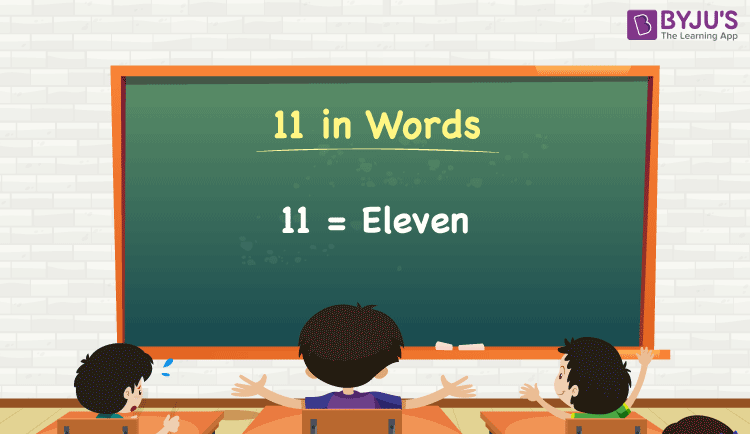# 11 in Words

11 in words can be written as Eleven. Using a place value chart, we can easily derive the number 11 in words. If you have 11 coins, you can say, “I have Eleven coins with me”. Here, 11 is a cardinal number since it denotes some specific quantity.

 11 in words: Eleven Eleven in numerical form: 11

In this article, you will learn how to write the number 11 in words, and some interesting facts about 11.

11 in English Words

We generally write numbers in words using the English alphabet. So, we can read 11 using the words in English as “Eleven”.## How to Write 11 in Words?

11 is a two-digit number, so let’s make a place value chart with two columns as shown below.

 Tens Ones 1 1

Here, the digit at the tens place is 1 and the units place is 1. So, let us expand these digits as per the place values, and combine the results to get the final number.

1 × Tens + 1 × Ones

= 1 × 10 + 1 × 1

= 10 + 1

= 11

= Eleven

Therefore, 11 in words = Eleven.

Interesting way of writing 11 in words:

1 = One

10 = Ten

10 + 1 = 11

11 = Eleven

Ten + One = Eleven

### Facts About the Number 11

11 is a natural number that is the successor of 10 and the predecessor of 12.

11 in words – Eleven

Is 11 an odd number? – Yes

Is 11 an even number? – No

Is 11 a composite number? – No

Is 11 a prime number? – Yes

Is 11 a perfect square number? – No

Is 11 a perfect cube number? – No

### 11 to 20 in Words

 11 in words = Eleven 16 in words = Sixteen 12 in words = Twelve 17 in words = Seventeen 13 in words = Thirteen 18 in words = Eighteen 14 in words = Fourteen 19 in words = Nineteen 15 in words = Fifteen 20 in words = Twenty

21 in words = Twenty-one

40 in words = Forty

110 in words = One hundred ten

111 in words = One hundred eleven

1100 in words = One thousand and hundred (or) Eleven hundred

1111 in words = One thousand one hundred and eleven

## Frequently Asked Questions on 11 in Words

Q1

### How do you write 11 in English?

The spelling of 11 in English is Eleven, which means 11 in words can be expressed as Eleven.

Q2

### Write the value of 7 + 4 in words.

7 + 4 = 11
Seven + Four = Eleven
Therefore, 7 + 4 is equal to Eleven.

Q3

### How do you write Rs. 11 in words?

We can write Rs. 11 in words as Eleven rupees.

Test your Knowledge on 11 in Words